#### You may also like### Stats Statements

Are these statistical statements sometimes, always or never true? Or it is impossible to say?### Real-life Equations

Here are several equations from real life. Can you work out which measurements are possible from each equation?### Bent Out of Shape

An introduction to bond angle geometry.

# CSI: Chemical Scene Investigation

##### Age 16 to 18 Challenge Level:

The first part of this question is a gentle introduction to the drawing of isomers. Structural isomers are those which involve different arrangements of the carbon skeleton, and different placements of the functional groups. It should be noted that cis and trans isomers are classed as geometric isomers, and so are not included here: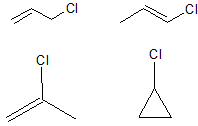The next part of the question is deliberately open ended. We have been able to find just over 30 different structures (maybe you can find more?), although it is important to note that many of these structures would be too unstable to be formed in the lab. However, this question is an exercise in logical structural drawing, rather than a detailed analysis of chemical stability.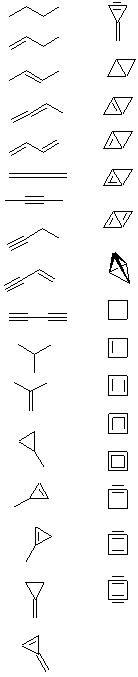The IR spectrum has three clear peaks:
~3100 cm$^-1$ broad peak correspond to -OH
~3300 cm$^-1$ corresponds to -NH
~1700 cm$^-1$ corresponds to C$=$O, rather than C$=$C since the absorption is SHARP.

The important point to note is that the peak at 3300cm$^-1$ is only single rather than a double absorption, which corresponds to an NH but NOT NH$_2$.

The zoomed IR spectrum shows an absorption at ~1670cm$^-1$, which indicates that the molecule contains an amide group. Thus because of the single peak at 3300cm$^-1$, this is a secondary amide: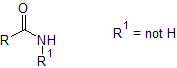The NRM spectrum has 6 peaks, which shows that there are 6 different carbon environments. Since six of the eight carbon atoms are present in a benzene ring, the location of the substituents around the ring must be: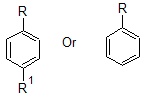From all the data so far, seven possible structures are: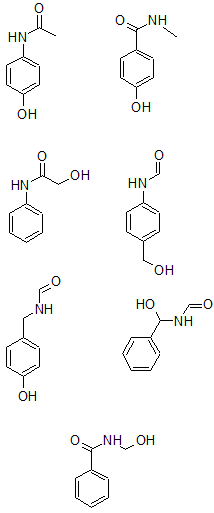The m/z of 58 corresponds to $[C_2H_4NO]^+$.
The m/z of 93 corresponds to $[C_6H_5O]^+$.

The additional information that the molecule was constructed from a nitro-aryl suggests that the nitrogen must be attached directly to the benzene ring. The only structure of the three remaining is: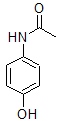This molecule is paracetamol.